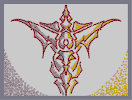### Silhouette In TwilightHover over the thumbnail for a full-size version.

Author SoldierGAMER author:soldiergamer bitesized n-art rated 2006-05-26 2006-05-26 4 by 57 people. \$Silhouette In Twilight#SoldierGAMER#none#00000000000000000000000000000000000000000000000000000000000000000000000000000000000000000000000000000000000000000000000000000000000000000000000000000000000000000000000000000000000000000000000000000000000000000000000000000000000000000000000000000000000000000000000000000000000000000000000000000000000000000000000000000000000000000000000000000000000000000000000000000000000000000000000000000000000000000000000000000000000000000000000000000000000000000000000000000000000000000000000000000000000000000000000000000000000000000000000000000000000000000000000000000000000000000000000000000000000000000000000000000000000000000000000000000000000000000000000000000000000000000000000000000000000000000000000000000000000000000|12^276,173!12^292,179!12^302,184!12^310,187!12^319,191!12^313,192!12^309,188!12^303,186!12^298,183!12^291,179!12^285,176!12^280,175!12^276,172!12^271,170!12^260,165!12^317,192!12^322,195!12^331,195!12^334,197!12^340,200!12^345,202!12^351,203!12^393,188!12^386,192!12^381,195!12^377,196!12^373,201!12^370,209!12^366,217!12^362,222!12^358,229!12^356,237!12^360,240!12^361,243!12^358,249!12^355,254!12^353,259!12^353,265!12^354,271!12^358,278!12^368,288!12^375,293!12^351,207!12^346,213!12^343,218!12^337,226!12^334,231!12^342,236!12^344,240!12^339,247!12^338,259!12^339,266!12^195,137!12^185,132!12^176,129!12^376,184!12^383,180!12^389,175!12^367,185!12^381,294!12^385,286!12^389,280!12^386,268!12^381,257!12^382,248!12^341,244!12^338,252!12^337,268!12^178,141!12^183,151!12^188,161!12^191,173!12^329,279!12^344,293!12^339,272!12^342,286!12^361,302!12^356,308!12^350,314!12^368,304!12^375,307!12^386,310!12^393,309!12^356,321!12^364,325!12^368,331!12^373,336!12^378,344!12^381,351!12^383,359!12^382,364!12^377,369!12^373,372!12^374,375!12^385,386!12^391,393!12^386,387!12^379,381!12^380,393!12^379,402!12^384,410!12^389,415!12^389,423!12^392,430!12^393,438!12^384,246!12^388,240!12^390,243!12^373,361!12^368,356!12^361,353!12^348,351!12^337,351!12^340,347!12^349,340!12^357,333!12^390,235!12^379,293!12^383,287!12^386,283!12^388,278!12^390,273!12^388,267!12^386,265!12^383,260!12^382,257!12^384,250!12^386,245!12^387,239!12^391,235!12^387,242!12^375,290!12^369,288!12^364,286!12^361,282!12^360,278!12^357,274!12^355,267!12^353,257!12^354,253!12^357,245!12^358,244!12^356,228!12^361,221!12^364,217!12^367,213!12^369,209!12^371,203!12^372,199!12^343,293!12^341,286!12^339,281!12^336,277!12^336,272!12^336,264!12^336,257!12^336,250!12^340,244!12^341,241!12^342,239!12^340,236!12^337,235!12^334,234!12^332,230!12^335,226!12^339,221!12^342,215!12^345,209!12^348,205!12^340,291!12^336,284!12^333,280!12^329,275!12^325,269!12^319,263!12^312,257!12^306,251!12^300,246!12^295,242!12^188,171!12^187,162!12^184,155!12^181,147!12^176,139!12^174,132!12^334,196!12^326,192!12^319,189!12^217,146!12^206,141!12^198,138!12^189,135!12^181,132!12^178,130!12^171,127!12^330,279!12^323,271!12^318,266!12^314,258!12^308,251!12^302,246!12^296,242!12^291,237!12^290,244!12^289,241!12^289,232!12^289,224!12^284,252!12^283,250!12^279,245!12^275,238!12^269,232!12^263,226!12^256,218!12^249,213!12^337,353!12^341,352!12^347,352!12^354,354!12^360,355!12^365,357!12^370,359!12^374,362!12^371,372!12^376,370!12^380,365!12^381,361!12^381,356!12^380,350!12^378,345!12^376,341!12^373,336!12^371,333!12^368,331!12^364,328!12^362,326!12^359,324!12^354,320!12^352,320!12^350,320!12^346,317!12^354,310!12^357,306!12^359,302!12^341,349!12^348,344!12^352,340!12^356,335!12^359,333!12^364,329!12^343,345!12^349,339!12^354,334!12^359,330!12^378,378!12^384,383!12^389,386!12^352,234!12^364,191!12^369,187!12^386,391!12^382,393!12^379,395!12^377,395!12^379,401!12^381,403!12^384,407!12^387,412!12^389,420!12^390,424!12^391,429!12^392,433!12^393,439!12^393,445!12^393,448!12^394,447!12^394,444!12^394,433!12^362,302!12^365,303!12^370,306!12^375,307!12^381,308!12^387,308!12^392,310!12^391,271!12^393,273!12^395,274!12^364,190!12^368,186!12^371,182!12^366,185!12^369,181!12^365,148!12^369,155!12^363,145!12^369,140!12^377,134!12^381,131!12^384,125!12^388,120!12^365,142!12^372,138!12^378,132!12^384,125!12^370,180!12^373,175!12^378,169!12^381,163!12^383,156!12^383,165!12^381,170!12^377,174!12^373,181!12^368,187!12^360,191!12^369,156!12^373,161!12^376,164!12^390,113!12^391,107!12^391,104!12^392,102!12^393,98!12^369,191!12^372,189!12^376,184!12^382,181!12^386,177!12^391,189!12^398,186!12^286,245!12^289,237!12^289,233!12^289,229!12^282,248!12^281,244!12^277,241!12^274,236!12^272,233!12^269,231!12^266,227!12^264,225!12^262,223!12^258,219!12^256,217!12^252,213!12^248,209!12^244,206!12^241,204!12^238,201!12^235,199!12^231,195!12^229,193!12^226,191!12^224,188!12^221,187!12^219,185!12^211,180!12^207,179!12^203,176!12^199,175!12^195,173!12^191,171!12^188,170!12^187,165!12^184,160!12^182,155!12^178,144!12^175,137!12^172,132!12^168,126!12^342,201!12^331,197!12^322,193!12^316,189!12^311,186!12^304,185!12^298,181!12^294,179!12^288,174!12^265,164!12^259,163!12^256,161!12^249,158!12^242,155!12^232,151!12^225,147!12^219,146!12^214,143!12^207,140!12^199,137!12^187,132!12^179,129!12^373,134!12^391,102!12^390,110!12^387,121!12^385,124!12^379,132!12^395,96!12^385,417!12^384,422!12^381,430!12^377,434!12^373,440!12^371,444!12^383,425!12^377,434!12^373,441!12^369,443!12^364,445!12^362,454!12^364,458!12^365,462!12^367,467!12^370,471!12^373,480!12^375,484!12^370,475!12^373,482!12^377,488!12^378,493!12^390,532!12^391,537!12^393,543!12^394,552!12^394,556!12^395,564!12^395,569!12^394,576!12^394,565!12^394,554!12^394,548!12^393,540!12^391,533!12^383,90!12^382,85!12^381,79!12^383,73!12^386,66!12^389,60!12^387,61!12^389,56!12^391,50!12^392,43!12^392,37!12^392,35!12^394,32!12^389,533!12^388,529!12^385,524!12^382,521!12^380,517!12^376,514!12^376,512!12^380,506!12^383,503!12^387,499!12^379,497!12^380,501!12^213,184!12^348,192!12^349,189!12^349,185!12^353,178!12^357,174!12^355,168!12^352,162!12^350,155!12^349,149!12^349,141!12^350,137!12^347,136!12^344,134!12^339,130!12^334,133!12^329,138!12^315,143!12^301,146!12^290,145!12^276,142!12^241,135!12^311,171!12^302,167!12^293,163!12^283,159!12^275,155!12^267,151!12^256,147!12^248,143!12^240,140!12^346,191!12^342,189!12^338,185!12^334,182!12^326,179!12^318,176!12^269,168!12^352,187!12^352,180!12^355,177!12^356,172!12^353,164!12^351,158!12^348,142!12^342,188!12^340,186!12^335,183!12^331,181!12^324,178!12^315,174!12^307,171!12^303,168!12^299,167!12^296,165!12^292,162!12^288,161!12^280,157!12^273,155!12^270,154!12^269,153!12^264,151!12^255,148!12^248,145!12^245,144!12^243,143!12^240,139!12^240,135!12^248,136!12^250,137!12^257,139!12^262,141!12^267,141!12^270,142!12^278,145!12^284,145!12^289,145!12^294,144!12^300,144!12^308,144!12^315,143!12^322,139!12^327,135!12^336,128!12^305,142!12^316,140!12^323,137!12^330,131!12^182,164!12^172,137!12^168,135!12^164,132!12^160,131!12^156,128!12^151,126!12^146,123!12^140,122!12^133,119!12^127,118!12^122,117!12^116,116!12^110,115!12^102,115!12^98,115!12^107,117!12^117,117!12^136,121!12^177,159!12^173,156!12^168,152!12^163,148!12^153,141!12^98,118!12^188,173!12^187,177!12^189,177!12^177,161!12^173,158!12^166,152!12^92,116!12^157,147!12^143,137!12^138,135!12^129,131!12^121,128!12^155,143!12^147,139!12^140,136!12^129,131!12^119,128!12^84,117!12^79,120!12^71,123!12^124,127!12^116,126!12^107,123!12^99,122!12^91,120!12^86,119!12^136,135!12^130,131!12^122,128!12^113,124!12^105,122!12^97,122!12^91,122!12^82,122!12^76,122!12^72,124!12^68,126!12^289,223!12^289,221!12^289,216!12^289,NaN!12^319,300!12^323,305!12^329,309!12^333,316!12^330,320!12^327,329!12^324,337!12^323,346!12^323,356!12^323,367!12^325,376!12^321,361!12^319,354!12^319,344!12^321,336!12^329,324!12^331,322!12^330,322!12^326,328!12^323,338!12^321,348!12^321,358!12^323,368!12^335,315!12^331,313!12^328,310!12^319,300!12^365,473!12^357,470!12^354,468!12^351,466!12^345,466!12^339,466!12^342,465!12^352,466!12^358,467!12^338,464!12^335,465!12^332,465!12^343,478!12^342,479!12^339,482!12^337,483!12^335,486!12^332,488!12^332,489!12^329,493!12^327,495!12^324,497!12^328,497!12^333,499!12^340,505!12^329,465!12^326,458!12^317,444!12^330,464!12^326,459!12^322,451!12^316,442!12^310,434!12^305,428!12^327,461!12^326,459!12^318,447!12^315,443!12^309,433!12^302,424!12^300,419!12^303,413!12^306,407!12^308,401!12^310,395!12^310,391!12^312,386!12^313,378!12^312,378!12^327,377!12^329,385!12^332,392!12^335,400!12^339,407!12^342,413!12^346,416!12^351,423!12^354,427!12^351,422!12^346,417!12^342,413!12^337,406!12^334,399!12^331,393!12^330,386!12^328,379!12^327,379!12^325,371!12^321,361!12^318,346!12^329,500!12^319,300!12^316,295!12^314,293!12^310,287!12^307,283!12^305,277!12^385,93!12^388,99!12^389,104!12^384,120!12^385,120!12^385,116!12^389,109!12^384,96!12^386,103!12^201,181!12^203,184!12^205,188!12^206,192!12^207,197!12^207,201!12^207,203!12^207,207!12^206,210!12^207,212!12^206,214!12^208,216!12^213,219!12^220,224!12^231,235!12^241,245!12^248,257!12^254,271!12^206,218!12^206,222!12^206,223!12^213,222!12^218,226!12^225,230!12^234,238!12^242,245!12^248,253!12^253,260!12^257,269!12^257,274!12^259,282!12^260,291!12^261,298!12^261,306!12^266,307!12^267,303!12^270,296!12^273,288!12^278,281!12^283,274!12^289,266!12^295,263!12^301,260!12^306,259!12^214,225!12^223,228!12^231,234!12^241,243!12^250,253!12^255,261!12^258,273!12^260,285!12^261,295!12^261,303!12^261,308!12^262,310!12^262,313!12^261,317!12^260,322!12^259,327!12^259,334!12^257,342!12^257,347!12^257,347!12^258,340!12^259,332!12^259,321!12^259,309!12^262,304!12^259,295!12^259,286!12^259,277!12^256,270!12^252,263!12^247,256!12^246,250!12^242,246!12^237,243!12^234,238!12^229,234!12^264,311!12^266,306!12^269,298!12^272,291!12^274,285!12^278,278!12^283,273!12^291,267!12^302,261!12^308,261!12^267,299!12^265,290!12^309,372!12^309,373!12^312,377!12^332,502!12^339,506!12^343,511!12^339,508!12^345,513!12^348,517!12^352,524!12^355,532!12^358,538!12^361,546!12^363,553!12^364,558!12^362,553!12^360,544!12^354,531!12^351,524!12^347,515!12^379,127!12^374,131!12^371,126!12^369,123!12^366,119!12^361,117!12^360,114!12^365,109!12^368,104!12^372,98!12^375,94!12^379,88!12^380,82!12^376,77!12^376,77!12^378,74!12^382,69!12^359,113!12^365,108!12^369,101!12^375,93!12^377,79!12^362,114!12^480,37712!12^500,179!12^490,184!12^482,187!12^473,191!12^479,192!12^483,188!12^489,186!12^494,183!12^501,179!12^507,176!12^512,175!12^516,172!12^521,170!12^532,165!12^475,192!12^470,195!12^461,195!12^458,197!12^452,200!12^447,202!12^441,203!12^399,188!12^406,192!12^411,195!12^415,196!12^419,201!12^422,209!12^426,217!12^430,222!12^434,229!12^436,237!12^432,240!12^431,243!12^434,249!12^437,254!12^439,259!12^439,265!12^438,271!12^434,278!12^424,288!12^417,293!12^441,207!12^446,213!12^449,218!12^455,226!12^458,231!12^450,236!12^448,240!12^453,247!12^454,259!12^453,266!12^597,137!12^607,132!12^616,129!12^416,184!12^409,180!12^403,175!12^425,185!12^411,294!12^407,286!12^403,280!12^406,268!12^411,257!12^410,248!12^451,244!12^454,252!12^455,268!12^614,141!12^609,151!12^604,161!12^601,173!12^463,279!12^448,293!12^453,272!12^450,286!12^431,302!12^436,308!12^442,314!12^424,304!12^417,307!12^406,310!12^399,309!12^436,321!12^428,325!12^424,331!12^419,336!12^414,344!12^411,351!12^409,359!12^410,364!12^415,369!12^419,372!12^418,375!12^407,386!12^401,393!12^406,387!12^413,381!12^412,393!12^413,402!12^408,410!12^403,415!12^403,423!12^400,430!12^399,438!12^408,246!12^404,240!12^402,243!12^419,361!12^424,356!12^431,353!12^444,351!12^455,351!12^452,347!12^443,340!12^435,333!12^402,235!12^413,293!12^409,287!12^406,283!12^404,278!12^402,273!12^404,267!12^406,265!12^409,260!12^410,257!12^408,250!12^406,245!12^405,239!12^401,235!12^405,242!12^417,290!12^423,288!12^428,286!12^431,282!12^432,278!12^435,274!12^437,267!12^439,257!12^438,253!12^435,245!12^434,244!12^436,228!12^431,221!12^428,217!12^425,213!12^423,209!12^421,203!12^420,199!12^449,293!12^451,286!12^453,281!12^456,277!12^456,272!12^456,264!12^456,257!12^456,250!12^452,244!12^451,241!12^450,239!12^452,236!12^455,235!12^458,234!12^460,230!12^457,226!12^453,221!12^450,215!12^447,209!12^444,205!12^452,291!12^456,284!12^459,280!12^463,275!12^467,269!12^473,263!12^480,257!12^486,251!12^492,246!12^497,242!12^604,171!12^605,162!12^608,155!12^611,147!12^616,139!12^618,132!12^458,196!12^466,192!12^473,189!12^575,146!12^586,141!12^594,138!12^603,135!12^611,132!12^614,130!12^621,127!12^462,279!12^469,271!12^474,266!12^478,258!12^484,251!12^490,246!12^496,242!12^501,237!12^502,244!12^503,241!12^503,232!12^503,224!12^508,252!12^509,250!12^513,245!12^517,238!12^523,232!12^529,226!12^536,218!12^543,213!12^455,353!12^451,352!12^445,352!12^438,354!12^432,355!12^427,357!12^422,359!12^418,362!12^421,372!12^416,370!12^412,365!12^411,361!12^411,356!12^412,350!12^414,345!12^416,341!12^419,336!12^421,333!12^424,331!12^428,328!12^430,326!12^433,324!12^438,320!12^440,320!12^442,320!12^446,317!12^438,310!12^435,306!12^433,302!12^451,349!12^444,344!12^440,340!12^436,335!12^433,333!12^428,329!12^449,345!12^443,339!12^438,334!12^433,330!12^414,378!12^408,383!12^403,386!12^440,234!12^428,191!12^423,187!12^406,391!12^410,393!12^413,395!12^415,395!12^413,401!12^411,403!12^408,407!12^405,412!12^403,420!12^402,424!12^401,429!12^400,433!12^399,439!12^399,445!12^399,448!12^398,447!12^398,444!12^398,433!12^430,302!12^427,303!12^422,306!12^417,307!12^411,308!12^405,308!12^400,310!12^401,271!12^399,273!12^397,274!12^428,190!12^424,186!12^421,182!12^426,185!12^423,181!12^427,148!12^423,155!12^429,145!12^423,140!12^415,134!12^411,131!12^408,125!12^404,120!12^427,142!12^420,138!12^414,132!12^408,125!12^422,180!12^419,175!12^414,169!12^411,163!12^409,156!12^409,165!12^411,170!12^415,174!12^419,181!12^424,187!12^432,191!12^423,156!12^419,161!12^416,164!12^402,113!12^401,107!12^401,104!12^400,102!12^399,98!12^423,191!12^420,189!12^416,184!12^410,181!12^406,177!12^401,189!12^394,186!12^506,245!12^503,237!12^503,233!12^503,229!12^510,248!12^511,244!12^515,241!12^518,236!12^520,233!12^523,231!12^526,227!12^528,225!12^530,223!12^534,219!12^536,217!12^540,213!12^544,209!12^548,206!12^551,204!12^554,201!12^557,199!12^561,195!12^563,193!12^566,191!12^568,188!12^571,187!12^573,185!12^581,180!12^585,179!12^589,176!12^593,175!12^597,173!12^601,171!12^604,170!12^605,165!12^608,160!12^610,155!12^614,144!12^617,137!12^620,132!12^624,126!12^450,201!12^461,197!12^470,193!12^476,189!12^481,186!12^488,185!12^494,181!12^498,179!12^504,174!12^527,164!12^533,163!12^536,161!12^543,158!12^550,155!12^560,151!12^567,147!12^573,146!12^578,143!12^585,140!12^593,137!12^605,132!12^613,129!12^419,134!12^401,102!12^402,110!12^405,121!12^407,124!12^413,132!12^397,96!12^407,417!12^408,422!12^411,430!12^415,434!12^419,440!12^421,444!12^409,425!12^415,434!12^419,441!12^423,443!12^428,445!12^430,454!12^428,458!12^427,462!12^425,467!12^422,471!12^419,480!12^417,484!12^422,475!12^419,482!12^415,488!12^414,493!12^402,532!12^401,537!12^399,543!12^398,552!12^398,556!12^397,564!12^397,569!12^398,576!12^398,565!12^398,554!12^398,548!12^399,540!12^401,533!12^409,90!12^410,85!12^411,79!12^409,73!12^406,66!12^403,60!12^405,61!12^403,56!12^401,50!12^400,43!12^400,37!12^400,35!12^398,32!12^403,533!12^404,529!12^407,524!12^410,521!12^412,517!12^416,514!12^416,512!12^412,506!12^409,503!12^405,499!12^413,497!12^412,501!12^579,184!12^444,192!12^443,189!12^443,185!12^439,178!12^435,174!12^437,168!12^440,162!12^442,155!12^443,149!12^443,141!12^442,137!12^445,136!12^448,134!12^453,130!12^458,133!12^463,138!12^477,143!12^491,146!12^502,145!12^516,142!12^551,135!12^481,171!12^490,167!12^499,163!12^509,159!12^517,155!12^525,151!12^536,147!12^544,143!12^552,140!12^446,191!12^450,189!12^454,185!12^458,182!12^466,179!12^474,176!12^523,168!12^440,187!12^440,180!12^437,177!12^436,172!12^439,164!12^441,158!12^444,142!12^450,188!12^452,186!12^457,183!12^461,181!12^468,178!12^477,174!12^485,171!12^489,168!12^493,167!12^496,165!12^500,162!12^504,161!12^512,157!12^519,155!12^522,154!12^523,153!12^528,151!12^537,148!12^544,145!12^547,144!12^549,143!12^552,139!12^552,135!12^544,136!12^542,137!12^535,139!12^530,141!12^525,141!12^522,142!12^514,145!12^508,145!12^503,145!12^498,144!12^492,144!12^484,144!12^477,143!12^470,139!12^465,135!12^456,128!12^487,142!12^476,140!12^469,137!12^462,131!12^610,164!12^620,137!12^624,135!12^628,132!12^632,131!12^636,128!12^641,126!12^646,123!12^652,122!12^659,119!12^665,118!12^670,117!12^676,116!12^682,115!12^690,115!12^694,115!12^685,117!12^675,117!12^656,121!12^615,159!12^619,156!12^624,152!12^629,148!12^639,141!12^694,118!12^604,173!12^605,177!12^603,177!12^615,161!12^619,158!12^626,152!12^700,116!12^635,147!12^649,137!12^654,135!12^663,131!12^671,128!12^637,143!12^645,139!12^652,136!12^663,131!12^673,128!12^708,117!12^713,120!12^721,123!12^668,127!12^676,126!12^685,123!12^693,122!12^701,120!12^706,119!12^656,135!12^662,131!12^670,128!12^679,124!12^687,122!12^695,122!12^701,122!12^710,122!12^716,122!12^720,124!12^724,126!12^503,223!12^503,221!12^503,216!12^503,NaN!12^473,300!12^469,305!12^463,309!12^459,316!12^462,320!12^465,329!12^468,337!12^469,346!12^469,356!12^469,367!12^467,376!12^471,361!12^473,354!12^473,344!12^471,336!12^463,324!12^461,322!12^462,322!12^466,328!12^469,338!12^471,348!12^471,358!12^469,368!12^457,315!12^461,313!12^464,310!12^473,300!12^427,473!12^435,470!12^438,468!12^441,466!12^447,466!12^453,466!12^450,465!12^440,466!12^434,467!12^454,464!12^457,465!12^460,465!12^449,478!12^450,479!12^453,482!12^455,483!12^457,486!12^460,488!12^460,489!12^463,493!12^465,495!12^468,497!12^464,497!12^459,499!12^452,505!12^463,465!12^466,458!12^475,444!12^462,464!12^466,459!12^470,451!12^476,442!12^482,434!12^487,428!12^465,461!12^466,459!12^474,447!12^477,443!12^483,433!12^490,424!12^492,419!12^489,413!12^486,407!12^484,401!12^482,395!12^482,391!12^480,386!12^479,378!12^480,378!12^465,377!12^463,385!12^460,392!12^457,400!12^453,407!12^450,413!12^446,416!12^441,423!12^438,427!12^441,422!12^446,417!12^450,413!12^455,406!12^458,399!12^461,393!12^462,386!12^464,379!12^465,379!12^467,371!12^471,361!12^474,346!12^463,500!12^473,300!12^476,295!12^478,293!12^482,287!12^485,283!12^487,277!12^407,93!12^404,99!12^403,104!12^408,120!12^407,120!12^407,116!12^403,109!12^408,96!12^406,103!12^591,181!12^589,184!12^587,188!12^586,192!12^585,197!12^585,201!12^585,203!12^585,207!12^586,210!12^585,212!12^586,214!12^584,216!12^579,219!12^561,235!12^551,245!12^544,257!12^538,271!12^586,218!12^586,222!12^586,223!12^579,222!12^574,226!12^567,230!12^558,238!12^550,245!12^544,253!12^539,260!12^535,269!12^535,274!12^533,282!12^532,291!12^531,298!12^531,306!12^526,307!12^525,303!12^522,296!12^519,288!12^514,281!12^509,274!12^503,266!12^497,263!12^491,260!12^486,259!12^578,225!12^569,228!12^561,234!12^551,243!12^542,253!12^537,261!12^534,273!12^532,285!12^531,295!12^531,303!12^531,308!12^530,310!12^530,313!12^531,317!12^532,322!12^533,327!12^533,334!12^535,342!12^535,347!12^535,347!12^534,340!12^533,332!12^533,321!12^533,309!12^530,304!12^533,295!12^533,286!12^533,277!12^536,270!12^540,263!12^545,256!12^546,250!12^550,246!12^555,243!12^558,238!12^563,234!12^528,311!12^526,306!12^523,298!12^520,291!12^518,285!12^514,278!12^509,273!12^501,267!12^490,261!12^484,261!12^525,299!12^527,290!12^483,372!12^483,373!12^480,377!12^460,502!12^453,506!12^449,511!12^453,508!12^447,513!12^444,517!12^440,524!12^437,532!12^434,538!12^431,546!12^429,553!12^428,558!12^430,553!12^432,544!12^438,531!12^441,524!12^445,515!12^413,127!12^418,131!12^421,126!12^423,123!12^426,119!12^431,117!12^432,114!12^427,109!12^424,104!12^420,98!12^417,94!12^413,88!12^412,82!12^416,77!12^416,77!12^414,74!12^410,69!12^433,113!12^427,108!12^423,101!12^417,93!12^415,79!12^430,114!3^375,553!3^371,543!3^365,533!3^360,523!3^355,512!3^348,504!3^339,497!3^347,490!3^364,506!3^370,499!3^336,456!3^331,447!3^324,437!3^318,429!3^311,421!3^316,410!3^319,400!3^320,389!3^268,280!3^266,268!3^262,258!3^258,249!3^252,241!3^245,235!3^237,227!3^229,219!3^219,215!3^218,206!3^215,194!3^276,272!3^283,264!3^274,260!3^372,352!3^363,346!3^350,345!3^363,341!3^397,529!3^392,521!3^387,513!3^391,507!3^394,513!3^387,491!3^384,481!3^380,471!3^375,461!3^370,453!3^380,447!3^384,438!3^382,459!3^362,418!3^354,412!3^345,402!3^341,393!3^337,383!3^333,372!3^333,365!3^362,409!3^356,404!3^349,395!3^341,378!3^366,400!3^357,393!3^349,386!3^334,308!3^323,298!3^316,286!3^311,278!3^319,275!3^325,285!3^329,295!3^329,264!3^323,256!3^314,247!3^305,241!3^300,236!3^296,223!3^305,234!3^327,250!3^333,245!3^322,241!3^314,235!3^280,236!3^280,224!3^275,227!3^268,215!3^261,209!3^253,200!3^244,194!3^236,188!3^226,180!3^217,173!3^210,169!3^199,165!3^194,155!3^189,144!3^203,152!3^210,162!3^235,179!3^226,171!3^220,164!3^215,155!3^346,455!3^356,460!3^345,448!3^337,440!3^322,421!3^332,432!3^358,499!3^380,566!3^379,561!3^174,153!3^164,144!3^155,136!3^145,132!3^132,126!3^344,178!3^335,173!3^321,166!3^328,169!3^313,163!3^301,157!3^289,154!3^262,145!3^274,149!3^282,153!3^327,159!3^327,153!3^335,140!3^305,151!3^315,151!3^326,148!3^377,151!3^371,149!3^388,168!3^391,159!3^394,169!3^378,117!3^367,111!3^375,118!3^382,276!3^376,280!3^368,277!3^361,266!3^363,256!3^368,268!3^377,273!3^366,233!3^372,226!3^381,204!3^382,225!3^386,200!3^378,208!3^376,219!3^381,215!3^393,89!3^390,78!3^391,68!3^23,301!3^260,569!3^33,321!3^244,561!3^226,551!3^206,536!3^52,366!3^39,340!3^61,382!3^126,473!3^189,526!3^170,513!3^152,499!3^138,488!3^112,459!3^101,444!3^89,428!3^75,404!3^241,574!3^223,567!3^204,559!3^181,547!3^164,535!3^148,524!3^133,511!3^116,493!3^97,476!3^86,458!3^76,446!3^62,426!3^49,408!3^35,386!3^26,364!3^191,569!3^175,561!3^154,550!3^134,534!3^114,522!3^96,505!3^77,483!3^62,468!3^46,445!3^32,416!3^161,568!3^141,563!3^128,552!3^109,537!3^93,526!3^78,513!3^65,500!3^56,489!3^43,472!3^32,457!3^128,571!3^115,561!3^94,547!3^77,536!3^62,526!3^51,517!3^40,504!3^33,488!3^44,496!3^59,510!3^107,569!3^92,565!3^80,556!3^62,547!3^52,539!3^42,530!3^35,517!3^83,571!3^67,566!3^53,557!3^42,549!3^34,537!3^59,571!3^30,553!3^34,568!3^41,563!3^109,550!3^145,546!3^112,510!3^80,497!3^95,492!3^59,450!3^32,437!3^46,426!3^36,401!3^210,572!3^279,574!3^130,500!3^112,477!3^75,432!3^46,392!3^60,415!3^37,374!3^28,352!3^151,536!3^171,550!3^189,559!3^78,471!3^149,513!3^193,543!3^173,530!3^217,556!3^49,461!3^54,478!3^72,460!3^69,483!3^58,440!3^62,398!3^98,466!3^89,445!3^42,357!3^30,504!3^29,530!3^30,476!3^148,577!3^124,543!3^182,577!0^420,564!0^421,553!0^423,541!0^424,533!0^428,522!0^423,551!0^425,540!0^428,532!0^430,526!0^434,517!0^440,510!0^447,502!0^454,497!0^448,493!0^441,484!0^448,490!0^441,485!0^445,492!0^445,500!0^441,512!0^401,525!0^406,518!0^409,515!0^403,509!0^401,508!0^406,490!0^410,483!0^411,474!0^413,466!0^416,459!0^419,455!0^411,442!0^417,449!0^422,453!0^420,459!0^417,468!0^409,444!0^437,460!0^445,458!0^454,458!0^460,454!0^464,445!0^468,436!0^467,439!0^474,432!0^478,429!0^479,424!0^481,423!0^479,423!0^480,418!0^476,414!0^473,405!0^472,397!0^474,391!0^455,450!0^461,438!0^465,430!0^466,420!0^468,407!0^471,421!0^434,417!0^428,407!0^427,406!0^430,408!0^430,404!0^438,398!0^442,391!0^448,385!0^452,377!0^456,368!0^461,368!0^457,377!0^454,386!0^451,394!0^449,399!0^441,412!0^442,402!0^445,400!0^437,410!0^450,386!0^421,351!0^427,349!0^434,348!0^430,341!0^427,339!0^421,350!0^435,347!0^462,303!0^467,294!0^470,284!0^471,278!0^479,273!0^480,281!0^474,288!0^466,297!0^475,278!0^517,576!0^665,473!0^766,301!0^414,272!0^421,268!0^430,257!0^422,264!0^428,257!0^414,277!0^418,283!0^421,280!0^428,273!0^428,264!0^423,275!0^415,277!0^413,279!0^418,282!0^428,231!0^422,228!0^413,226!0^411,219!0^421,226!0^417,222!0^414,214!0^412,205!0^410,200!0^411,223!0^410,217!0^409,206!0^423,226!0^419,221!0^412,209!0^408,203!0^404,199!0^402,165!0^401,160!0^405,171!0^400,156!0^414,154!0^418,150!0^420,149!0^413,146!0^436,408!0^443,403!0^450,390!0^476,422!0^476,406!0^469,422!0^474,406!0^480,416!0^473,405!0^470,414!0^467,430!0^458,451!0^442,510!0^416,121!0^420,115!0^422,113!0^418,110!0^415,116!0^399,89!0^401,83!0^404,76!0^402,74!0^400,68!0^461,245!0^467,246!0^475,245!0^480,240!0^488,234!0^493,229!0^494,224!0^464,249!0^464,259!0^464,265!0^468,257!0^473,251!0^482,245!0^490,238!0^495,232!0^508,264!0^516,262!0^526,254!0^501,262!0^526,255!0^538,244!0^542,239!0^550,231!0^559,223!0^564,217!0^527,254!0^532,250!0^561,219!0^569,211!0^573,204!0^576,195!0^513,268!0^521,274!0^525,280!0^525,284!0^525,271!0^526,264!0^520,267!0^525,263!0^546,236!0^555,230!0^575,208!0^578,199!0^572,220!0^576,216!0^577,198!0^515,215!0^525,209!0^539,202!0^551,189!0^563,179!0^569,174!0^574,167!0^580,158!0^587,146!0^596,144!0^601,142!0^597,150!0^593,156!0^510,215!0^512,220!0^514,228!0^514,232!0^518,219!0^532,206!0^542,201!0^527,213!0^524,216!0^516,228!0^514,233!0^546,198!0^561,189!0^565,185!0^572,176!0^578,168!0^584,161!0^591,153!0^587,167!0^594,160!0^599,149!0^594,163!0^597,164!0^454,142!0^457,146!0^457,155!0^456,163!0^455,170!0^452,175!0^448,179!0^453,176!0^462,169!0^469,166!0^481,161!0^488,157!0^498,155!0^503,154!0^510,152!0^518,149!0^469,171!0^484,161!0^482,159!0^468,155!0^464,153!0^464,147!0^469,156!0^466,166!0^466,149!0^474,154!0^482,154!0^490,154!0^474,162!0^470,164!0^463,148!0^601,144!0^588,157!0^582,165!0^569,179!0^564,182!0^518,227!0^512,225!0^511,232!0^620,147!0^627,142!0^634,137!0^642,135!0^648,132!0^656,127!0^664,124!3^127,525!3^121,509!3^106,496!3^126,484!3^106,484!3^102,517!3^87,541!3^75,527!3^65,537!3^90,516!3^87,483!3^31,426!3^31,448!3^32,468!3^28,395!3^29,378!3^28,408!3^44,417!3^49,380!3^28,340!3^27,328!3^69,417!3^81,418!3^44,484!3^41,456!3^43,437!3^27,515!3^26,570!3^45,573!3^71,548!3^66,558!3^51,528!3^68,513!3^43,515!3^53,500!3^30,544!3^101,559!3^101,536!3^152,557!3^129,564!3^98,573!3^116,574!3^160,524!3^87,507!3^76,503!3^64,493!3^170,574!3^151,568!3^163,547!3^135,547!3^118,531!3^200,575!3^229,577!3^202,549!0^521,576!0^537,572!0^542,573!0^762,314!0^757,327!0^748,350!0^740,372!0^732,391!0^717,410!0^703,433!0^688,452!0^653,487!0^637,499!0^614,518!0^596,537!0^555,567!0^533,574!0^686,450!0^704,427!0^716,408!0^741,367!0^765,309!0^581,552!0^588,545!0^600,535!0^614,525!0^625,515!0^642,493!0^667,472!0^686,453!0^694,441!0^707,424!0^717,410!0^729,392!0^739,374!0^749,354!0^759,330!0^765,314!0^760,571!0^763,561!0^763,562!0^759,567!0^747,572!0^740,572!0^748,567!0^752,561!0^756,551!0^759,551!0^759,565!0^748,574!0^733,573!0^730,571!0^738,568!0^747,559!0^757,544!0^761,535!0^756,551!0^735,568!0^720,577!0^718,574!0^734,561!0^747,548!0^755,531!0^755,536!0^742,554!0^719,565!0^697,573!0^705,569!0^720,558!0^740,544!0^753,525!0^758,538!0^764,559!0^761,541!0^759,520!0^759,506!0^748,531!0^730,548!0^698,570!0^673,575!0^687,557!0^711,540!0^735,521!0^748,499!0^749,521!0^567,570!0^589,558!0^618,540!0^635,523!0^660,498!0^677,482!0^695,460!0^710,440!0^726,421!0^756,391!0^768,346!0^757,369!0^746,402!0^729,426!0^742,388!0^732,417!0^679,473!0^638,500!0^611,536!0^641,512!0^582,566!0^613,556!0^643,534!0^664,516!0^691,489!0^716,465!0^742,429!0^763,401!0^729,458!0^727,438!0^697,471!0^653,511!0^601,559!0^653,525!0^686,492!0^754,426!0^739,472!0^687,527!0^621,559!0^607,563!0^665,531!0^698,487!0^738,445!0^730,484!0^675,530!0^608,559!0^670,516!0^712,482!0^732,465!0^670,530!0^666,532!0^710,480!0^716,486!0^677,538!0^634,567!0^679,538!0^718,505!0^656,561!0^644,556!0^678,534!0^710,515!0^686,552!0^728,507!0^750,474!0^754,450!0^746,518!0^753,490!0^716,540!0^694,556!0^719,530!0^714,555!0^739,532!0^717,557!0^664,574!0^700,542!0^739,503!0^687,543!0^671,557!0^701,550!0^733,536!0^725,540!0^660,566!0^645,557!0^684,530!0^710,490!0^689,514!0^653,551!0^637,561!0^696,528!0^735,480!0^675,566!0^720,504!0^721,528!0^717,533!0^709,515!0^708,511!0^692,529!0^648,559!0^623,573!0^610,568!0^656,565!0^683,556!0^676,549!0^666,538!0^667,543!0^703,508!0^697,506!0^702,499!0^702,532!0^726,502!0^715,528!0^719,521!0^731,495!0^716,499!0^691,522!0^722,480!0^669,511!0^663,521!0^639,539!0^639,545!0^619,567!0^601,570!0^623,570!0^661,551!0^699,527!0^666,549!0^693,521!0^715,523!0^738,488!0^745,474!0^752,514!0^750,472!0^750,473!0^755,469!0^743,491!0^698,523!0^640,552!0^659,538!0^623,561!0^581,565!0^645,539!0^720,467!0^725,447!0^702,474!0^719,454!0^686,501!0^664,529!0^708,556!0^734,544!0^703,566!0^732,550!0^724,546!0^702,555!0^740,502!0^751,443!0^757,467!0^569,557!0^679,458!0^687,519!0^759,448!0^400,411!0^405,405!0^407,402!0^402,401!0^406,377!0^409,374!0^405,372!0^412,335!0^417,329!0^421,323!0^419,315!0^415,311!0^428,312!0^429,311!0^429,316!0^428,317!3^362,312!3^363,319!3^373,316!3^370,320!3^370,324!3^381,341!3^378,332!3^379,319!3^384,373!3^387,401!3^391,410!0^738,458!0^753,429!0^748,486!0^680,562!0^680,562!0^747,416!0^698,475!0^669,501!0^647,530!0^698,476!0^722,441!0^674,478!0^653,497!0^700,466!0^725,436!0^752,386!0^762,354!0^756,409!0^751,477!0^753,499!0^609,545!0^639,527!0^623,543!0^652,539# So, what you think about it?

Pages: (0)

4

### reminds me

of bayonette from that game robo something.
THere's a trophy of it in super smash brothers
hope u dont mind but iv set this as my desktop wallpaper. i love this sorta stuff! 6/5

### My New Map

See My New Crazy Map - http://numa.notdot.net/map/48159

### ahhm,

This is N, not paint dotnet or a white paper. He dont have to make a real-life-picture in ned (I dont misunderstand u, nemetacyst, I only say what I think). 4.5/5 :]

### i think

that this just plain looks cool, regardless of how the sun sets/rises etc.

### um...

well....on cartographs...or basically anything else...

west is left...east is right...your colors are backwards...
cuz last time i checked, the sun didnt go down in the east...
and thas shading...ug...it adds color, not any sort of direction...not only is the transition from color to not-color bad, but it doesnt even match the direction of light in many places...

dont get me wrong, i dont dislike it, your arts are getting better...but in my opinion, this does not deserve such a high rating...4 minimum(yes, minimum), but its place is not in the top page of arts...

### ........

.... And i'm don't understand too, why all told that my map's "so great, and beatyfull"....

### Hmmmm

... So, That silhouete of dragon, but i'm separate him parts...
Twinlight - that Sun on West and go down, And Darkness From East Up.
3d-ness that i'm try to show light and shadows on the picture.....

It's not a Japanesse anime!!! I'm Hate Anime!!!

p.s. And sorry my english, i'm rus.

### um...

silhouette of what?
what twilight?
am i really missing the 3-d ness of this???
more specifically, what 3d ness...the light is coming from at least 2 or 3 different directions if thats what you call shading...
wtf is this?!?!
is it a demented japanese anime airplane from hell?!?!?!
am i the only one whose confused here?!?!?!?!?!?!

i wont rate until i know what the hell is going on...

### wow

this is great art. like the 3Dness.
4,5/5

look at my N arts!

### ............

..... And if u think that i'm lamer u get a big mistake!

### Hmmm

I'm said Thx beacose not see it before. It my 3-th map, and i'm don't need "Edit" before. U must set the rating to image craft...

### 4/5

He said thanks to Brttrx because Brttrx told him about the "Edit" button.

~Mosh

Omg lol wtf? :D

Brttrx - Thx

### Okay,

this is pretty good, but just not up to par with Dragon_moons most recent dragon, which conatains so much more detail and uses the whole screen. I looked at your maps, and you are definitly getting better. I'm sure you will be able to make something up to par with Dragon Moon sometime soon.
until then,
4/5

### omg wtf bbq hax

awesome. you're the next Dragon Moon

### And by the way,

this is phenomenal work. 5/5 for sure. Screw those dragons.

### Just look next to the name of the map

when ur signed in, you see "edit", click that, make spelling corrections, submit, return to map

### That's teh awesomezorz!

5/5 for your hard work.
And please dont type in all caps....

### SORRY !!

SORRY MY ENGLISH !!
NOT THUNK - THINK !!
IT'S A MISPRINT !!

### Sweet

Looks pretty awsome in the thumbnail.